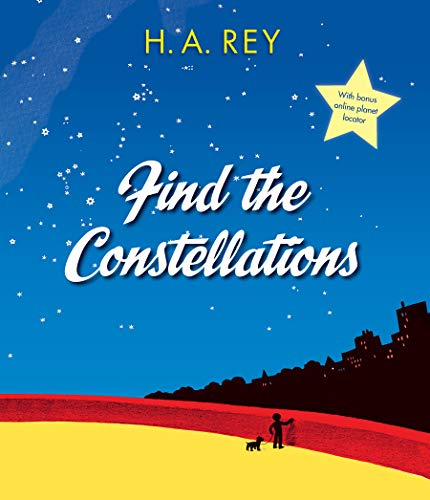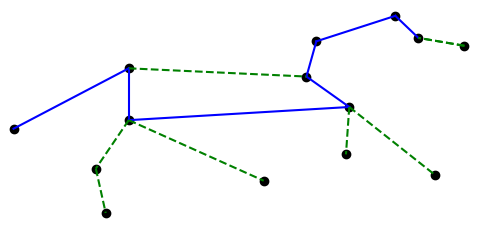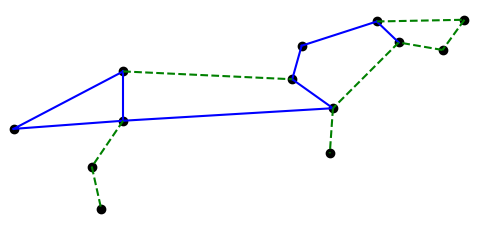Until yesterday, I was only aware of the traditional assignment of stars to constellations. In the comments to yesterday’s post I learned that H. A. Rey, best known for writing the Curious George books, came up with a new way of viewing the constellations in 1952, adding stars and connecting lines in order to make the constellations look more like their names. For example, to make Leo look more like a lion.The International Astronomical Union (IAU) makes beautiful star charts of the constellations, and uses Rey’s conventions, sorta.

This post will look at the example of Leo, from the IAU chart and from Rey’s book Find The Constellations.

(I wonder whether the ancients also added stars to what we received as the traditional versions of constellations. Maybe they didn’t consciously notice the other stars. Or maybe they did, but only saw the need to record the brightest stars, something like the way Hebrew only recorded the consonants of words.)

Here is the IAU star chart for Leo, cropped to just show the constellation graph. (The white region is Leo-as-region and the green lines are Leo-as-graph.)Rey’s version of Leo is a little different. Here is my attempt to reproduce Rey’s version from page 9 of Find the Constellations.And for comparison, here’s my reproduction of the IAU version.The solid blue lines are traditional. The dashed green lines were added by Rey and the IAU respectively.

Here is the Python code that produced the two images. Star names and coordinates are explained in the previous post.

```# data from https://en.wikipedia.org/wiki/List_of_stars_in_Leo
import matplotlib.pyplot as plt

# star coordinates
δ = (11 + 14/60, 20 + 41/60)
β = (11 + 49/60, 14 + 34/60)
θ = (11 + 14/60, 15 + 26/60)
α = (10 +  8/60, 11 + 58/60)
η = (10 +  7/60, 16 + 46/60)
γ = (10 + 20/60, 19 + 51/60)
ζ = (10 + 17/60, 23 + 25/60)
μ = ( 9 + 53/60, 26 +  0/60)
ε = ( 9 + 46/60, 23 + 46/60)
κ = ( 9 + 25/60, 26 + 11/60)
λ = ( 9 + 32/60, 22 + 58/60)
ι = (11 + 24/60, 10 + 32/60)
σ = (11 + 21/60,  6 +  2/60)
ο = ( 9 + 41/60,  9 + 54/60)
ρ = (10 + 33/60,  9 + 18/60)

k = -20 # reverse and scale horizontal axis

def plot_stars(ss):
for s in ss:
plt.plot(k*s, s, 'ko')

def join(s0, s1, style, color):
plt.plot([k*s0, k*s1], [s0, s1], style, color=color)

def draw_iau():
plot_stars([δ,β,θ,α,η,γ,ζ,μ,ε,κ,λ,ι,σ])

join(δ, β, '-', 'b')
join(β, θ, '-', 'b')
join(θ, η, '-', 'b')
join(η, γ, '-', 'b')
join(γ, ζ, '-', 'b')
join(ζ, μ, '-', 'b')
join(μ, ε, '-', 'b')
join(δ, θ, '-', 'b')

join(θ, ι, '--', 'g')
join(ι, σ, '--', 'g')
join(δ, γ, '--', 'g')
join(ε, η, '--', 'g')
join(μ, κ, '--', 'g')
join(κ, λ, '--', 'g')
join(λ, ε, '--', 'g')
join(η, α, '--', 'g')

def draw_rey():
plot_stars([δ,β,θ,α,η,γ,ζ,μ,ε,λ,ι,σ, ρ,ο])

join(δ, β, '-', 'b')
# join(β, θ, '-', 'b')
join(θ, η, '-', 'b')
join(η, γ, '-', 'b')
join(γ, ζ, '-', 'b')
join(ζ, μ, '-', 'b')
join(μ, ε, '-', 'b')
join(δ, θ, '-', 'b')

join(θ, ι, '--', 'g')
join(ι, σ, '--', 'g')
join(δ, γ, '--', 'g')
join(λ, ε, '--', 'g')
join(η, α, '--', 'g')
join(λ, ε, '--', 'g')
join(θ, ρ, '--', 'g')
join(η, ο, '--', 'g')

```

## 2 thoughts on “Adding stars to constellations”

1. Jonathan

Not that I’m expecting a matplotlib script rendering these, but others who like constellation maps might enjoy the star atlas written by the 10th century astronomer al-Sufi, especially the version created for the 14th century ruler Ulugh Beg.

The IAU has (at least) one image from it on their site: Pegasus (https://www.iau.org/public/images/detail/iau1603b/).

The Bibliothèque nationale de France hosts the full text, behind a sorta complicated interface: https://portail.biblissima.fr/en/ark:/43093/mdata3fbdf7732fe8deba3cda349dda73fdc14925dfad

If you can figure out how to leaf through the text, you will see that it includes tables for the star positions, for those who can read medieval Arabic. Also, relevant to John’s question, is the fact that there aren’t *any* lines between any stars. The question “when did the joining lines appear?” deepens!

2. Hi, John. I’ve never read Rey’s “Find the Constellations.” His most important book is “The Stars: A New Way to See Them,” which is the one published in 1952 and has been in continuous publication ever since. His version of Leo in that classic book is much closer to the IAU version. You can see it on Wikipedia here: https://en.wikipedia.org/wiki/H._A._Rey#/media/File:Leo_constellation_map_visualization.PNG

According to Wikipedia, “Find the Constellations” was a simplified version for children, apparently published two years later. Maybe Rey learned city children were having a hard time tracing some of his figures with its dimmer starts?